# Absorption models

##### April 20, 2020
using Pumas, Plots


# Introduction

In this tutorial, we will cover the following absorption models

• first order

• zero-order

• two parallel first-order

• first-order + zero-order simultaneously

• Weibull absorption

• Erlang absorption

• Transit absorption (WIP)

## Generating the population

sd = DosageRegimen(100, time=0)
choose_covariates() = (Wt = rand(55:80),dose=100)
sd_with_covariates = Population(map(i -> Subject(id=i,evs=sd,cvs=choose_covariates()),1:10))

Population
Subjects: 10
Covariates: Wt, dose


For a more indepth tutorial, please see the tutorial on generating and simulation populations

For simplicity, we will use a 1-compartment distribution system with additional absorption compartment(s) as required. Note that we are not simulating with residual error in these examples, but that can be easily added

## first-order absorption

foabs = @model begin

@param begin
tvcl ∈ RealDomain(lower=0)
tvv ∈ RealDomain(lower=0)
tvka ∈ RealDomain(lower=0)
Ω ∈ PDiagDomain(3)
end

@random begin
η ~ MvNormal(Ω)
end

@covariates Wt

@pre begin
CL = tvcl*(Wt/70)^0.75*exp(η)
V  = tvv*(Wt/70)*exp(η)
Ka = tvka*exp(η)
end

@dynamics begin
Depot'   = -Ka*Depot
Central' =  Ka*Depot - Central*CL/V
end

@derived begin
conc = Central/V
end

end

PumasModel
Parameters: tvcl, tvv, tvka, Ω
Random effects: η
Covariates: Wt
Dynamical variables: Depot, Central
Derived: conc
Observed: conc


and below are the set of parameters for this model

param = (
tvcl = 5, tvv = 20, tvka = 1,
Ω =Diagonal([0.04,0.04,0.04]),
)

(tvcl = 5, tvv = 20, tvka = 1, Ω = [0.04 0.0 0.0; 0.0 0.04 0.0; 0.0 0.0 0.0
4])


We can now use the data and model with parameters to simulate a first-order absorption profile

obs = simobs(foabs, sd_with_covariates, param, obstimes=0:1:48)
foplot = plot(obs, title =  "First-order absorption")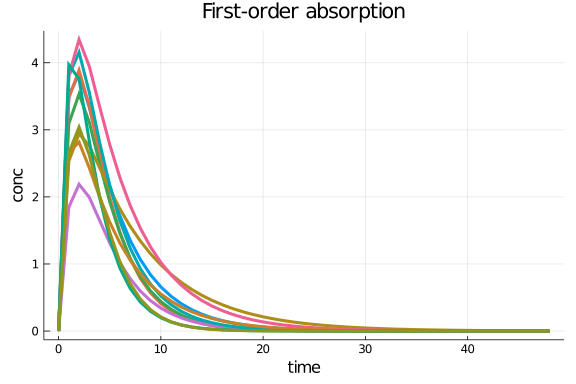## zero-order absorption

zoabs = @model begin
@param begin
tvcl ∈ RealDomain(lower=0)
tvv ∈ RealDomain(lower=0)
tvduration ∈ RealDomain(lower=0)
Ω ∈ PDiagDomain(3)
end

@random begin
η ~ MvNormal(Ω)
end

@covariates Wt

@pre begin
CL = tvcl*(Wt/11)^0.75*exp(η)
V  = tvv*(Wt/11)*exp(η)
duration =  (Central = tvduration*exp(η),)
end

@dynamics begin
Central' =  - Central*CL/V
end

@derived begin
conc = Central/V
end

end

PumasModel
Parameters: tvcl, tvv, tvduration, Ω
Random effects: η
Covariates: Wt
Dynamical variables: Central
Derived: conc
Observed: conc


and below are the set of parameters for this model

param = (
tvcl = 0.792, tvv = 13.7, tvduration = 5,
Ω =Diagonal([0.04,0.04,0.04]),
)

(tvcl = 0.792, tvv = 13.7, tvduration = 5, Ω = [0.04 0.0 0.0; 0.0 0.04 0.0;
0.0 0.0 0.04])


Notes:

1. Notice in the model above that there is no additional "absorption" compartment. We are

directly dosing into the central compartment as an infusion.This is a common trick used to model zero-order absorption profiles

1. Since we are deling with infusion, we have to set the rate data item in DosageRegimen to be < 0

We can now use the data and model with parameters to simulate a zero-order absorption profile

sd = DosageRegimen(100, time=0, rate=-2)
choose_covariates() = (Wt = rand(55:80),dose=100)
sd_with_covariates = Population(map(i -> Subject(id=i,evs=sd,cvs=choose_covariates()),1:10))

Population
Subjects: 10
Covariates: Wt, dose

obs = simobs(zoabs, sd_with_covariates, param, obstimes=0:0.1:48)
zoplot = plot(obs, title =  "Zero-order absorption")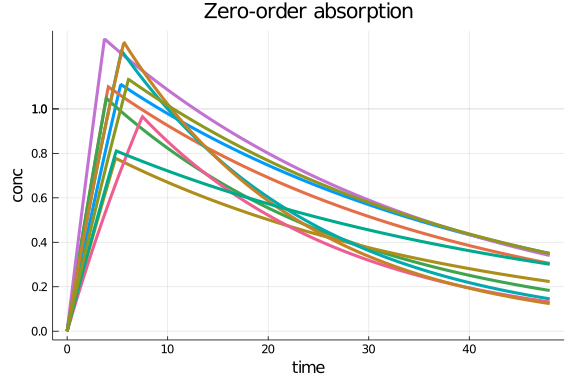## mixed zero+first order absorption models

fozoabs = @model begin

@param begin
tvcl ∈ RealDomain(lower=0)
tvv ∈ RealDomain(lower=0)
tvka ∈ RealDomain(lower=0)
tvduration ∈ RealDomain(lower=0)
tvbio ∈ RealDomain(lower=0)
tvlag ∈ RealDomain(lower=0)
Ω ∈ PDiagDomain(2)
end

@random begin
η ~ MvNormal(Ω)
end

@covariates Wt

@pre begin
CL = tvcl*(Wt/70)^0.75*exp(η)
V  = tvv*(Wt/70)*exp(η)
Ka = tvka
duration = (Central = tvduration,)
bioav = (Depot = tvbio, Central = 1-tvbio)
lags = (Depot = tvlag,)
end

@dynamics begin
Depot'   = -Ka*Depot
Central' =  Ka*Depot - Central*CL/V
end

@derived begin
conc = Central/V
end

end

PumasModel
Parameters: tvcl, tvv, tvka, tvduration, tvbio, tvlag, Ω
Random effects: η
Covariates: Wt
Dynamical variables: Depot, Central
Derived: conc
Observed: conc


and below are the set of parameters for this model

param = (
tvcl = 5, tvv = 50, tvka = 1.2, tvduration = 2, tvbio = 0.5, tvlag = 2,
Ω = Diagonal([0.04, 0.04]),
)

(tvcl = 5, tvv = 50, tvka = 1.2, tvduration = 2, tvbio = 0.5, tvlag = 2, Ω
= [0.04 0.0; 0.0 0.04])


Notes:

1. In the mixed order case, each subject will have two rows for dosing, the first-order one into the depot compartment

and the zero-order one into the central compartment.

1. Hence we see two specifications of dosing in using DosageRegimen below.

2. DosageRegimen can also combine multiple DosageRegimen's together, in this case, first and zero order

3. In this example, zero-order dosing occurs first over a 2 hour duration and the first hour process starts

after a lag of 2 hours.

We can now use the data and model with parameters to simulate a simultaneous first- and zero-order absorption profile

sd_fo = DosageRegimen(100, time=0, cmt=1, rate = 0, evid=1)
sd_zo = DosageRegimen(100, time=0, cmt=2, rate = -2, evid=1)
sd_fo_zo = DosageRegimen(sd_fo,sd_zo)

choose_covariates() = (Wt = rand(55:80),dose=100)
sd_with_covariates = Population(map(i -> Subject(id=i,evs=sd_fo_zo,cvs=choose_covariates()),1:10))

Population
Subjects: 10
Covariates: Wt, dose

obs = simobs(fozoabs, sd_with_covariates, param, obstimes=0:0.1:24)
fozoplot = plot(obs, title = "mixed zero+first order absorption")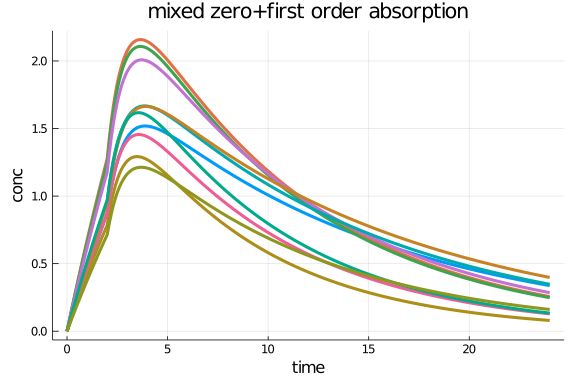## Two parallel first-order absorptions

two_parallel_foabs = @model begin

@param begin
tvcl ∈ RealDomain(lower=0)
tvv ∈ RealDomain(lower=0)
tvka1 ∈ RealDomain(lower=0)
tvka2 ∈ RealDomain(lower=0)
tvlag ∈ RealDomain(lower=0)
tvbio ∈ RealDomain(lower=0)
Ω ∈ PDiagDomain(6)
end

@random begin
η ~ MvNormal(Ω)
end

@covariates Wt

@pre begin
CL = tvcl*(Wt/70)^0.75*exp(η)
V  = tvv*(Wt/70)*exp(η)
Ka1 = tvka1*exp(η)
Ka2 = tvka2*exp(η)
lags = (SR = tvlag*exp(η),)
bioav = (IR = tvbio*exp(η), SR = 1-tvbio*exp(η))
end

@dynamics begin
IR'   = -Ka1*IR
SR'   = -Ka2*SR
Central' =  Ka1*IR + Ka2*SR - Central*CL/V
end

@derived begin
conc = Central/V
end

end

PumasModel
Parameters: tvcl, tvv, tvka1, tvka2, tvlag, tvbio, Ω
Random effects: η
Covariates: Wt
Dynamical variables: IR, SR, Central
Derived: conc
Observed: conc


and below are the set of parameters for this model

param = (
tvcl = 5, tvv = 50, tvka1 = 0.8, tvka2 = 0.6, tvlag = 5, tvbio = 0.5,
Ω =Diagonal([0.04,0.04,0.36,0.36,0.04,0.04]),
)

(tvcl = 5, tvv = 50, tvka1 = 0.8, tvka2 = 0.6, tvlag = 5, tvbio = 0.5, Ω =
[0.04 0.0 … 0.0 0.0; 0.0 0.04 … 0.0 0.0; … ; 0.0 0.0 … 0.04 0.0; 0.0 0.0 …
0.0 0.04])


We can now use the data and model with parameters to simulate a first-order absorption profile

fo_1 = DosageRegimen(100,cmt=1,time=0)
fo_2 = DosageRegimen(100,cmt=2,time=0)
parallel_fo = DosageRegimen(fo_1,fo_2)
parallel_fo_with_covariates = Population(map(i -> Subject(id=i,evs=parallel_fo,cvs=choose_covariates()),1:10))
obs = simobs(two_parallel_foabs, parallel_fo_with_covariates, param, obstimes=0:1:48)
parallel_fo_plot = plot(obs, title =  "Two Parallel First-order absorption")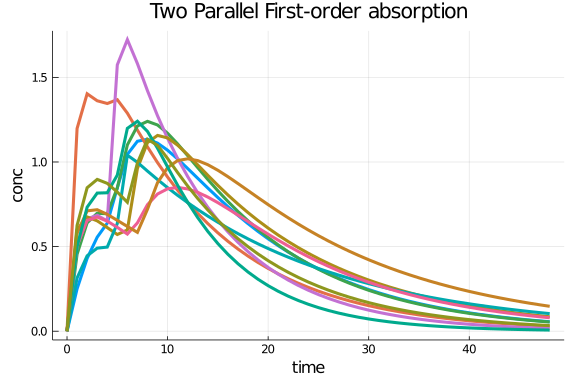## Weibull-Type Absorption

wb_abs = @model begin

@param begin
tvcl ∈ RealDomain(lower=0)
tvv ∈ RealDomain(lower=0)
tvka1 ∈ RealDomain(lower=0)
tvγ₁ ∈ RealDomain(lower=0)
Ω ∈ PDiagDomain(4)
end

@random begin
η ~ MvNormal(Ω)
end

@covariates Wt

@pre begin
CL = tvcl*(Wt/70)^0.75*exp(η)
V  = tvv*(Wt/70)*exp(η)
Ka1 = tvka1*exp(η)
γ₁ = tvγ₁*exp(η)
# Weibull function
wb = 1-exp((-(Ka1*t)^γ₁))
end

@dynamics begin
Depot'   = -wb*Depot
Central' =  wb*Depot - Central*CL/V
end

@derived begin
conc = Central/V
end

end

PumasModel
Parameters: tvcl, tvv, tvka1, tvγ₁, Ω
Random effects: η
Covariates: Wt
Dynamical variables: Depot, Central
Derived: conc
Observed: conc


and below are the set of parameters for this model

param = (
tvcl = 5, tvv = 50,tvka1 = 0.4, tvγ₁ = 4,
Ω =Diagonal([0.04,0.04,0.36,0.04]),
)

(tvcl = 5, tvv = 50, tvka1 = 0.4, tvγ₁ = 4, Ω = [0.04 0.0 0.0 0.0; 0.0 0.04
0.0 0.0; 0.0 0.0 0.36 0.0; 0.0 0.0 0.0 0.04])


We can now use the data and model with parameters to simulate a weibull absorption profile

wb_reg = DosageRegimen(100,cmt=1,time=0)
wb_reg_with_covariates = Population(map(i -> Subject(id=i,evs=wb_reg,cvs=choose_covariates()),1:10))
obs = simobs(wb_abs,wb_reg_with_covariates, param, obstimes=0:1:48)
wb_plot = plot(obs, title =  "Weibull absorption")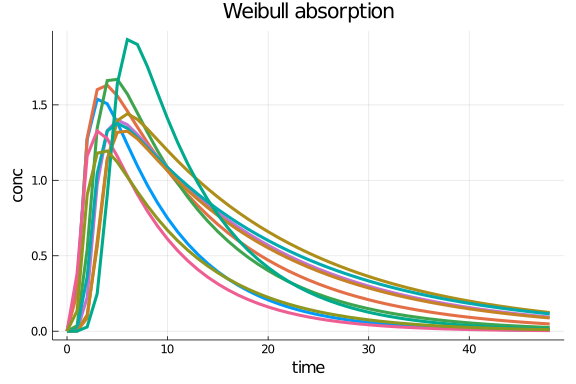## Erlang absorption

erlangabs = @model begin
@param begin
tvcl ∈ RealDomain(lower=0)
tvv ∈ RealDomain(lower=0)
tvktr ∈ RealDomain(lower=0)
tvq ∈ RealDomain(lower=0)
tvvp ∈ RealDomain(lower=0)
Ω ∈ PSDDomain(4)
end

@random begin
η ~ MvNormal(Ω)
end

@covariates Wt

@pre begin
CL = tvcl*(Wt/70)^0.75*exp(η)
Vc  = tvv*(Wt/70)*exp(η)
Ktr = tvktr*exp(η)
Q  = tvq*(Wt/70)^0.75*exp(η)
Vp  = tvvp*(Wt/70)
K  = CL/Vc
K21 = Q/Vp
K12 = Q/Vc
N = 5 # number of compartments
end

@dynamics begin
Depot' = -Ktr*Depot
DELA1' = Ktr*Depot-Ktr*DELA1
DELA2' = Ktr*DELA1-Ktr*DELA2
DELA3' = Ktr*DELA2-Ktr*DELA3
DELA4' = Ktr*DELA3-Ktr*DELA4
DELA5' = Ktr*DELA4-Ktr*DELA5
Central' = Ktr*DELA5 - Central*K + K21*Periph -K12*Central
Periph' = - K21*Periph + K12*Central
end

@derived begin
conc = @. Central/Vc
end

end

PumasModel
Parameters: tvcl, tvv, tvktr, tvq, tvvp, Ω
Random effects: η
Covariates: Wt
Dynamical variables: Depot, DELA1, DELA2, DELA3, DELA4, DELA5, Central, P
eriph
Derived: conc
Observed: conc


and below are the set of parameters for this model

param = (
tvcl = 27, tvv = 77.4, tvktr = 7.74, tvq = 23.7, tvvp = 55.6,
Ω = diagm(0 => [0.09,0.22,0.10,0.52])
)

(tvcl = 27, tvv = 77.4, tvktr = 7.74, tvq = 23.7, tvvp = 55.6, Ω = [0.09 0.
0 0.0 0.0; 0.0 0.22 0.0 0.0; 0.0 0.0 0.1 0.0; 0.0 0.0 0.0 0.52])


We can now use the data and model with parameters to simulate a erlang absorption profile

erlang_reg = DosageRegimen(100,cmt=1,time=0)
erlang_reg_with_covariates = Population(map(i -> Subject(id=i,evs=erlang_reg,cvs=choose_covariates()),1:10))
obs = simobs(erlangabs,erlang_reg_with_covariates, param, obstimes=0:1:24)
erlang_plot = plot(obs, title =  "Erlang absorption")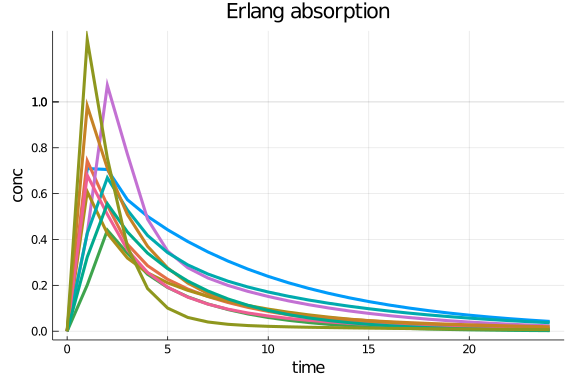using PumasTutorials
PumasTutorials.tutorial_footer(WEAVE_ARGS[:folder],WEAVE_ARGS[:file])

ERROR: KeyError: key :folder not found